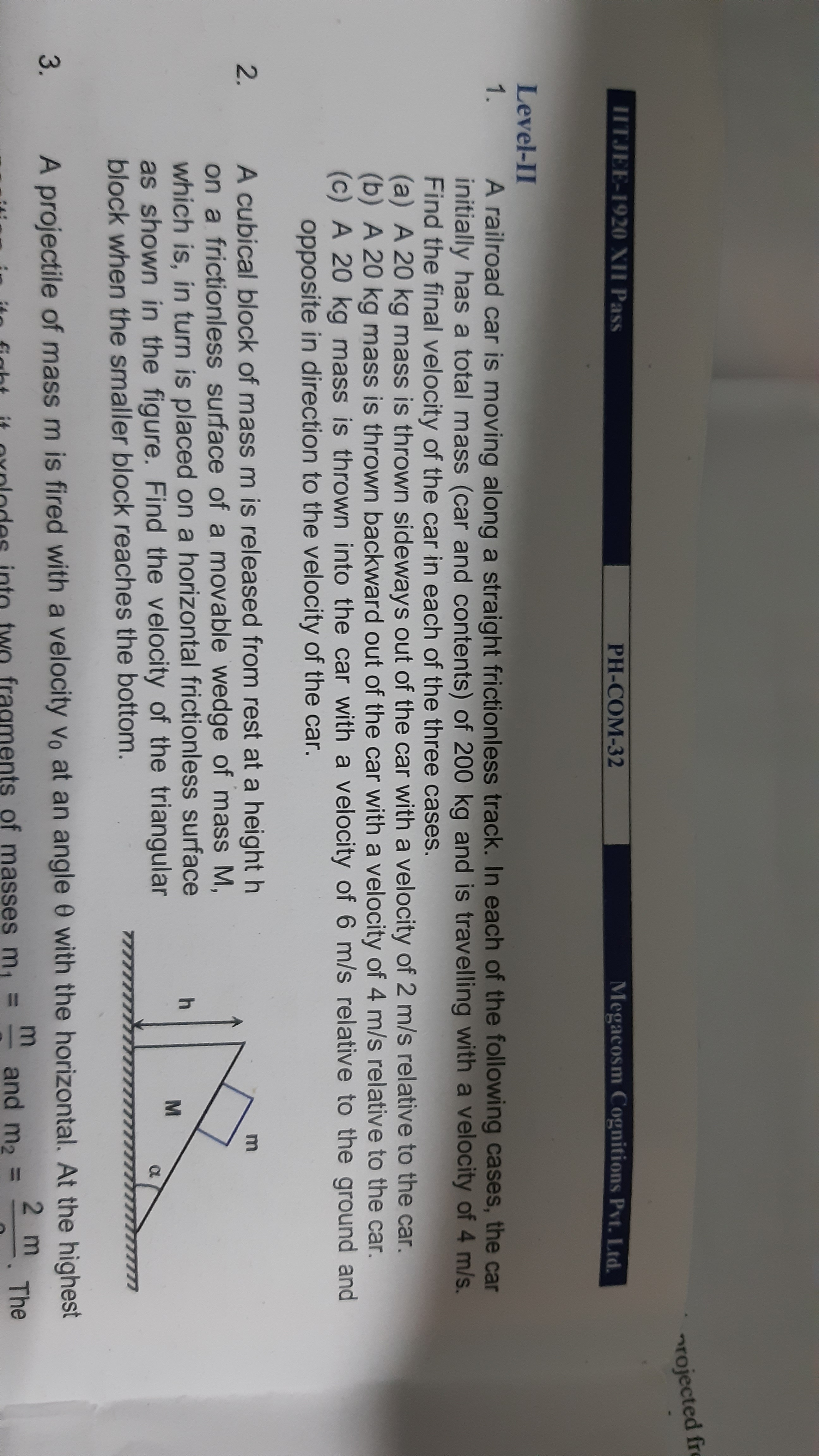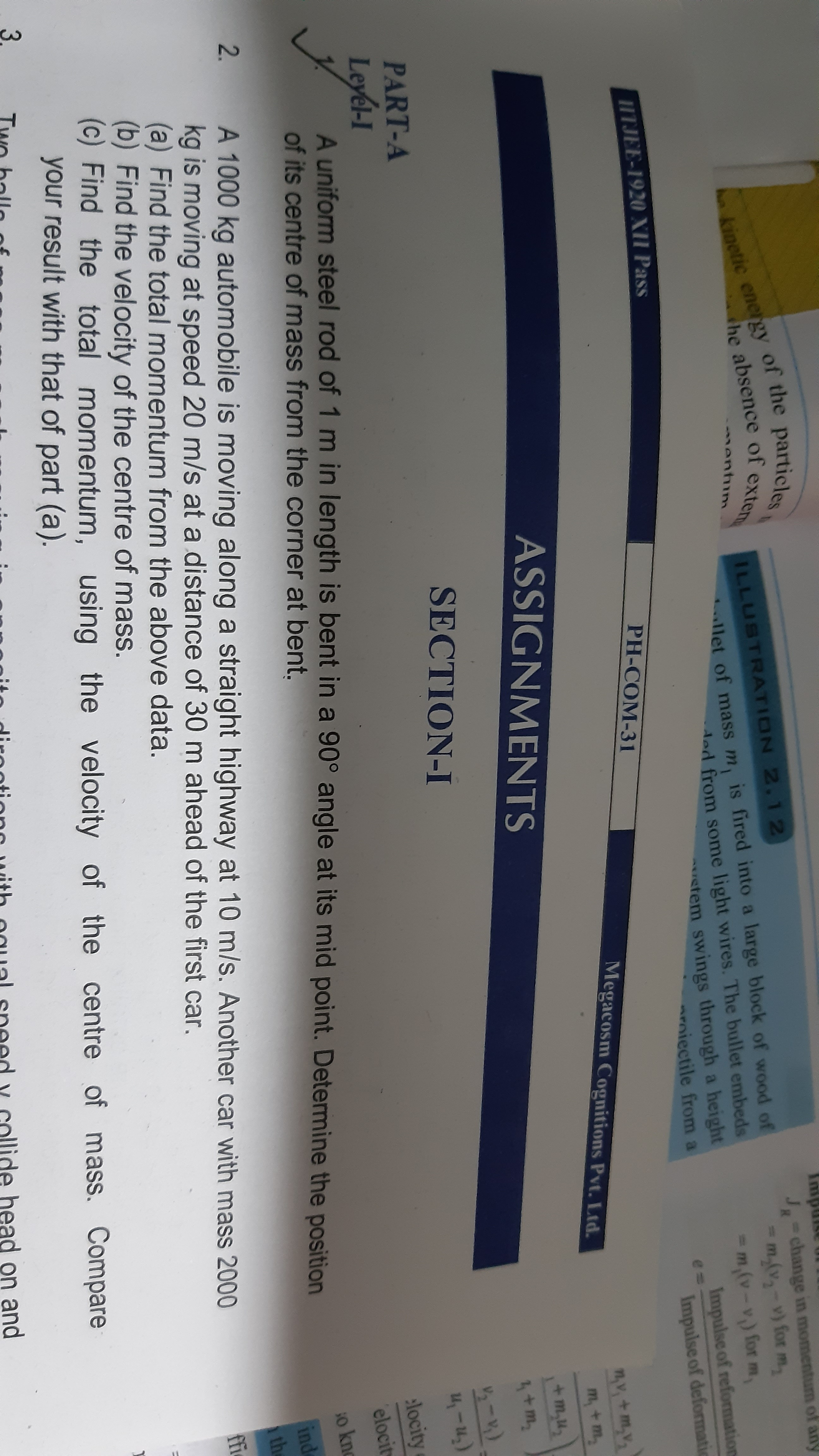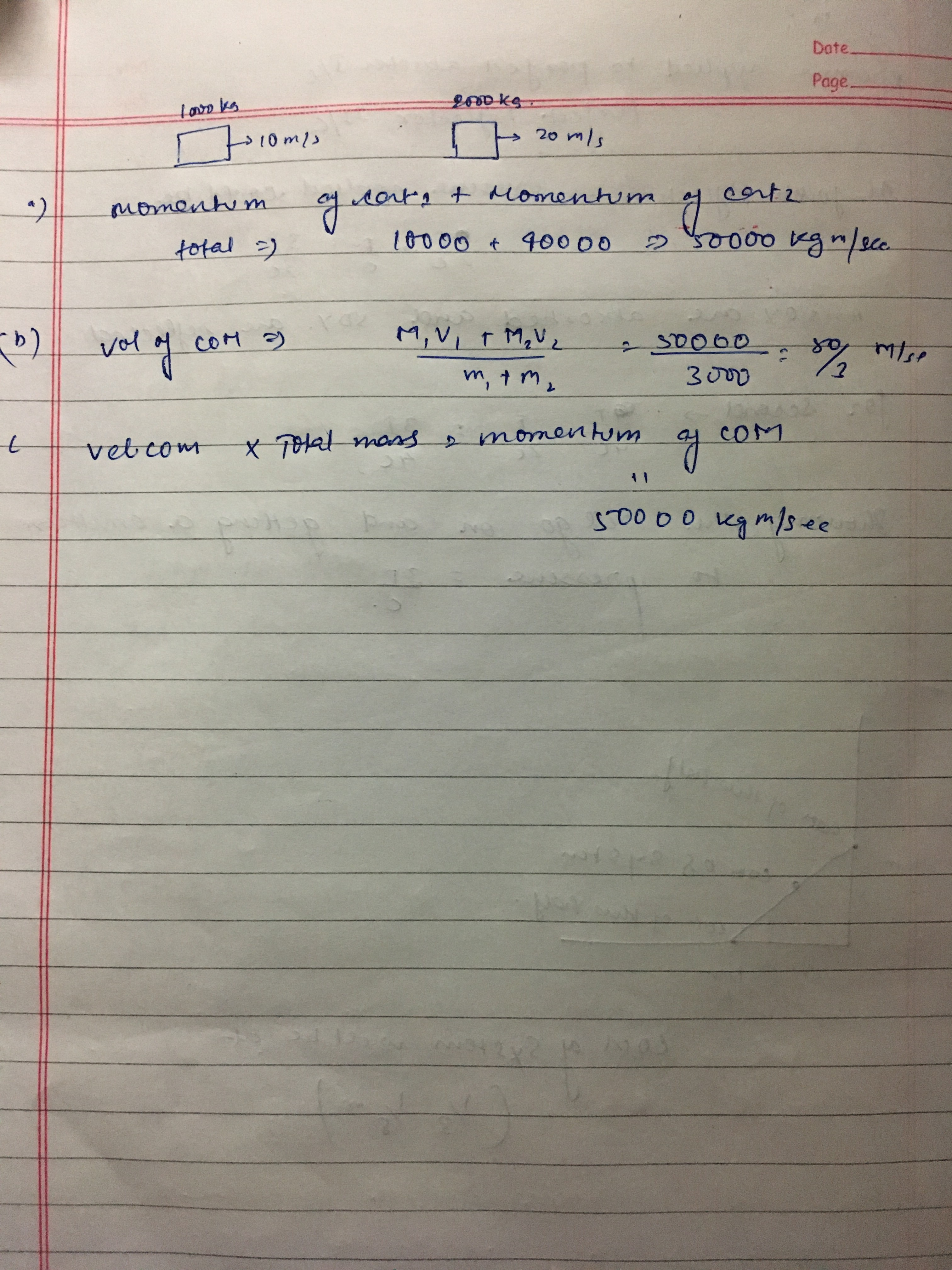# Doubt from momentum

Question no 2 level 1,question1 level 2For L1 q2
That seems very simple question , all you have to do is Vcom = m1v1+m2v2/m1+m2
Where exactly are you facing problem innthat question ?

What about a and c part i got b part

Part a ) total momentum = M1v1 + M2V2
C) total momentum =( M1+M2 ) Vcom

1 Like

What about level 2 question 1

For all questions of level 2 q1, all you have to do is conserve the momentum before which is = 200 * 4 along x in all the cases
And then assume new velocity of railroad car to be v and then write it’s new momentum = 800

Level 1 question 21 Like